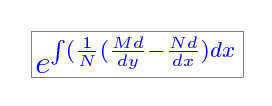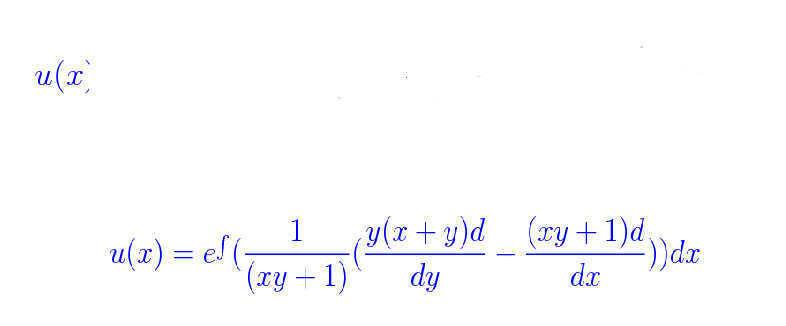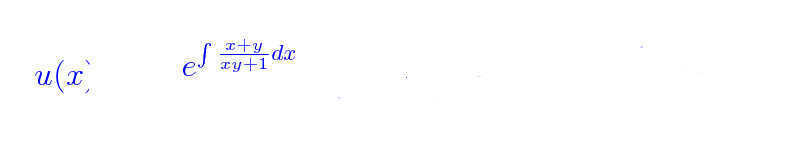# Special Integrating Factors

1. solve the problem first finding an integrating factor of susceptible form.
y(x+y)dx+(xy+1)dy=0

## Homework Equations

form: M(x,y)dx+N(x,y)dy=0
intigrating factor: eint(1/n(dm/dy-dndx)dx

## The Attempt at a Solution

u(x)=eint(1/(xy+1)(y(x+y)d/dy-(xy+1)d/dx)dx
this reduces to
eint((x+y)/(xy+1))dx

This is where I need help. my integration is not good. I know that if I can solve for the integrating factor then I can solve for the equation because it will be in exact form.

Thanks for any help.

## Answers and Replies

HallsofIvy
Science Advisor
Homework Helper
1. solve the problem first finding an integrating factor of susceptible form.
y(x+y)dx+(xy+1)dy=0

## Homework Equations

form: M(x,y)dx+N(x,y)dy=0
intigrating factor: eint(1/n(dm/dy-dndx)dx

## The Attempt at a Solution

u(x)=eint(1/(xy+1)(y(x+y)d/dy-(xy+1)d/dx)dx
This makes no sense to me. Your integrand is a function of x and y but you are integrating with respect to x so the exponent will be a function of y only yet you say that equals a function of x!

this reduces to
eint((x+y)/(xy+1))dx

This is where I need help. my integration is not good. I know that if I can solve for the integrating factor then I can solve for the equation because it will be in exact form.

Thanks for any help.

1. solve the problem first finding an integrating factor of susceptible form.
y(x+y)dx+(xy+1)dy=0

## Homework Equations

form: M(x,y)dx+N(x,y)dy=0
intigrating factor:## The Attempt at a Solution

( Please excuse the u(x) remnants i'm leaning how to use the white boar editor.)[/B]this reduces to ( Please excuse the u(x) reminets i'm leaning how to use the white boar editor.)This is where I need help. my integration is not good. I know that if I can solve for the integrating factor then I can solve for the equation because it will be in exact form.

Thanks for any help.
edited to make it easier to read

Halsofivy, you are right u(x) should be in terms of x. the way it works out in the book examples is y gets factored out while solving for the intigal. leaving only a function of x.